Private GMAT Tutoring in NYC & Online

A basket contains 5 apples, of which 1 is spoiled and the rest are good. If Henry is to select 2 apples from the basket simultaneously and at random, what is the possibility that the 2 apples selected will include the spoiled apple?

(A) 1/5
(B) 3/10
(C) 2/5
(D) 1/2
(E) 3/5

If two of the four expressions x+y, x+5y, x-y, and 5x-y are chosen at random, what is the probability that their product will be of the form of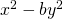, where b is an integer?

(A) 1/2

(B) 1/3

(C) 1/4

(D) 1/5

(E) 1/6

Be super comfortable with difference of squares. You're almost guaranteed to need it on your GMAT. You need to know it forwards, backwards, and upside-down. There isn't much content you need to know for the GMAT quant but in order to be consistently successful so that you can go in there on test day and snag your 700+ score you need complete fluency with that limited content. On this one we're looking for a product of two terms that will produce difference of squares. So we need something in the format: (x+y(x-y). There's only one pairing that gives us the right format. Now you have to figure out what that means in terms of probability. In general, probability is specific scenario/total scenarios. You can calculate the total and the specific scenarios using the slot method. How many teams of 2 can you make from four things? (4*3)/(1*2) = 6. So that's your denominator. And then there's only one team that works, the pairing of (x + y)(x - y). So that leaves you with 1/6. E. Detailed diagrams below and an in depth video explantation here: If two of the four expressions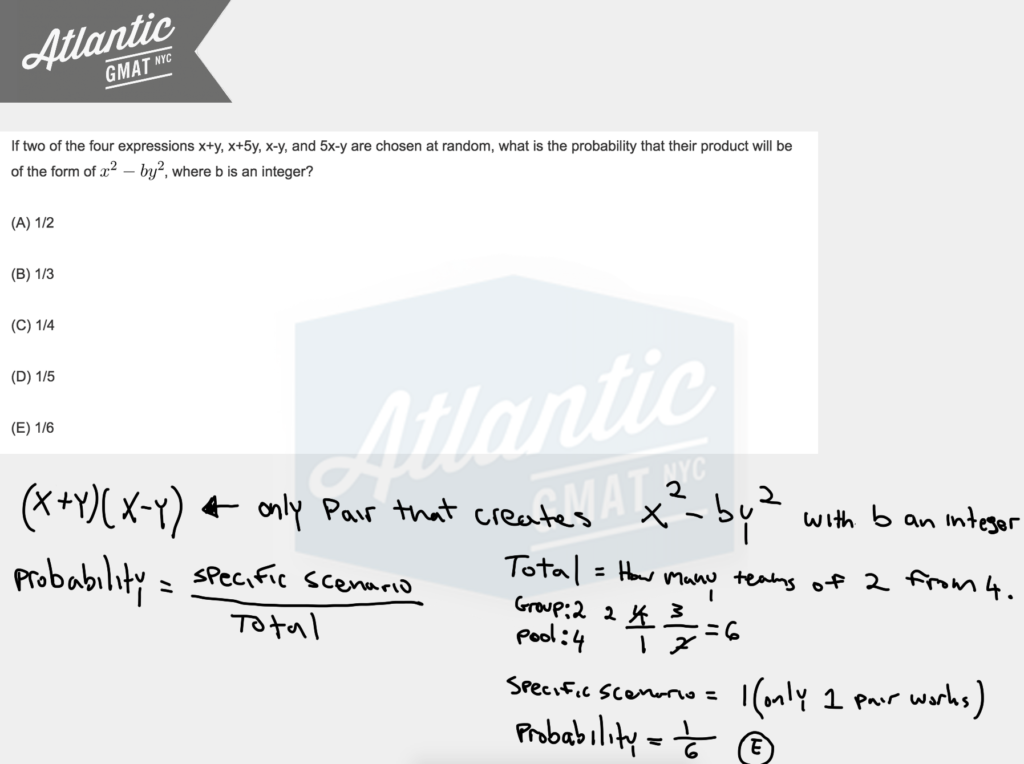You also can think about this in terms of straight probability and say: 2 choices our of 4 and then 1 choice out of 3. 2/4 * 1/3 = 2/12 = 1/6. Comment with any questions or additions. Happy studies!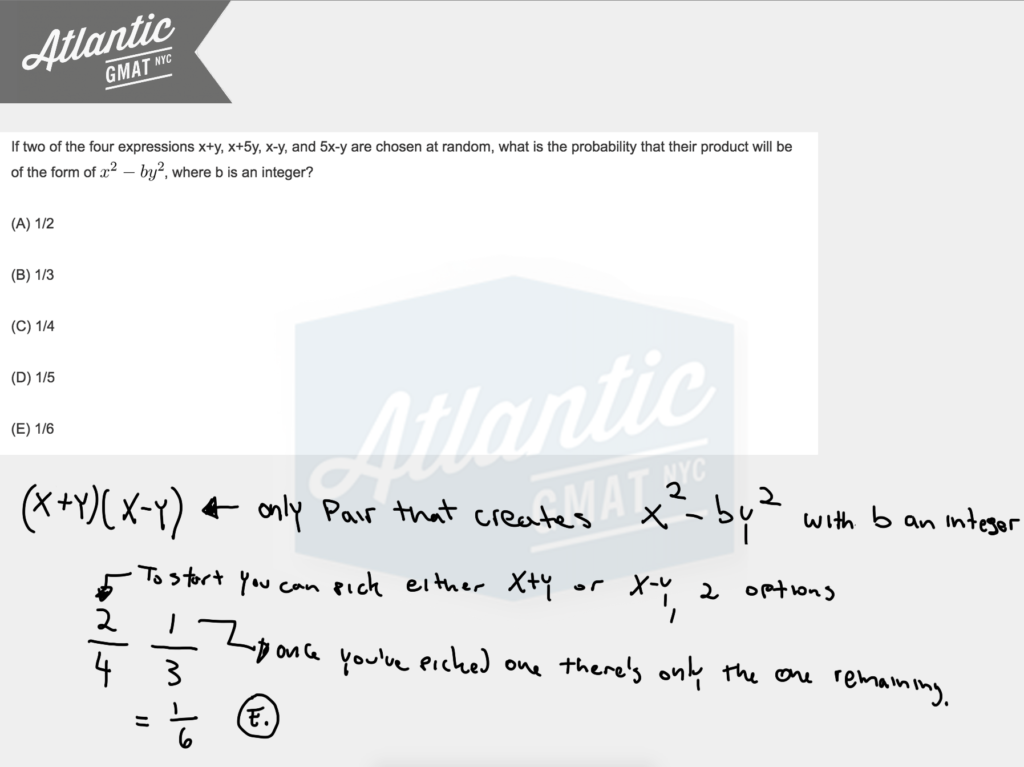# GMAT Question of the Day - Data Sufficiency - Probability

Roy has a bowl with 12 red and j blue marbles. If Roy picks two random marbles out of the bowl it is more likely that he will pick one of each color than only two blue marbles. Are there an equal number of red and blue marbles in the bowl?

(1) j > 11

(2) The chance of picking two blue marbles is greater than 5/23

## GMAT Question of the Day Solution

On GMAT Data Sufficiency questions take your time to understand the given information. Avoid rushing into the statements. In this case you need to unravel this statement: If Roy picks two random marbles out of the bowl it is more likely that he will pick one of each color than only two blue marbles. This is critical. Now you might be thinking that this question is very open ended because you don't know how many blue marbles you have but the given information severely limits the options. The number of blue marbles must be less than or equal to 25. Here's the math to calculate that.

This is based on this statement: If Roy picks two random marbles out of the bowl it is more likely that he will pick one of each color than only two blue marbles.

j/(12+j) * (j-1)/(12+j-1) *(2!/2!)  < j/(12+j)*12/(12+j-1)*(2!/(1!*1!))

j<25

Statement (1) Could be a Yes, 12, or a no with anything greater than 12. Statement (2) give you the same information but in a slightly different way. We need to know whether we have 12 blue marbles so just try plugging in 11 marbles or 12 marbles see which one (or maybe both) agrees with the information in the statement. We can see that when picking 11 you get exactly 5/23. So there must be more than 11 blue marbles. That is the same info as statement 1. You could have the same number of marbles 12 and 12 or you could have more blue marbles, up to 24.

# GMAT Question of the Day - Problem Solving - Probability

Dan will go on vacation starting Monday morning. If on any day of his vacation Dan's boss calls him, Dan must return to work that same evening. If on any given day there is a 20% chance that Dan's boss will call him, what is the probability that Dan will return to work on Thursday evening?

A. .0896

B. .0872

C. .1012

D. .1024

E. .1128

## GMAT Question of the Day Solution

In GMAT tutoring I'm always talking about staying organized. The people who I work with on GMAT are probably sick of me saying: "stay organized". But hey - organization is the key to a great GMAT score. On probability questions it can help to make a map of what you are calculating before you put the actual numbers in. This can help you plan the question. You can see an example of a map in the attached diagram. Also - be smart with your arithmetic. Look for ways to simplify your calculations. You can see that the answers are in decimals so it will be smart to keep the denominators in powers of 10 so it is easy to calculate the decimal. You can also see that the numerator can be calculated in terms of powers of 2's.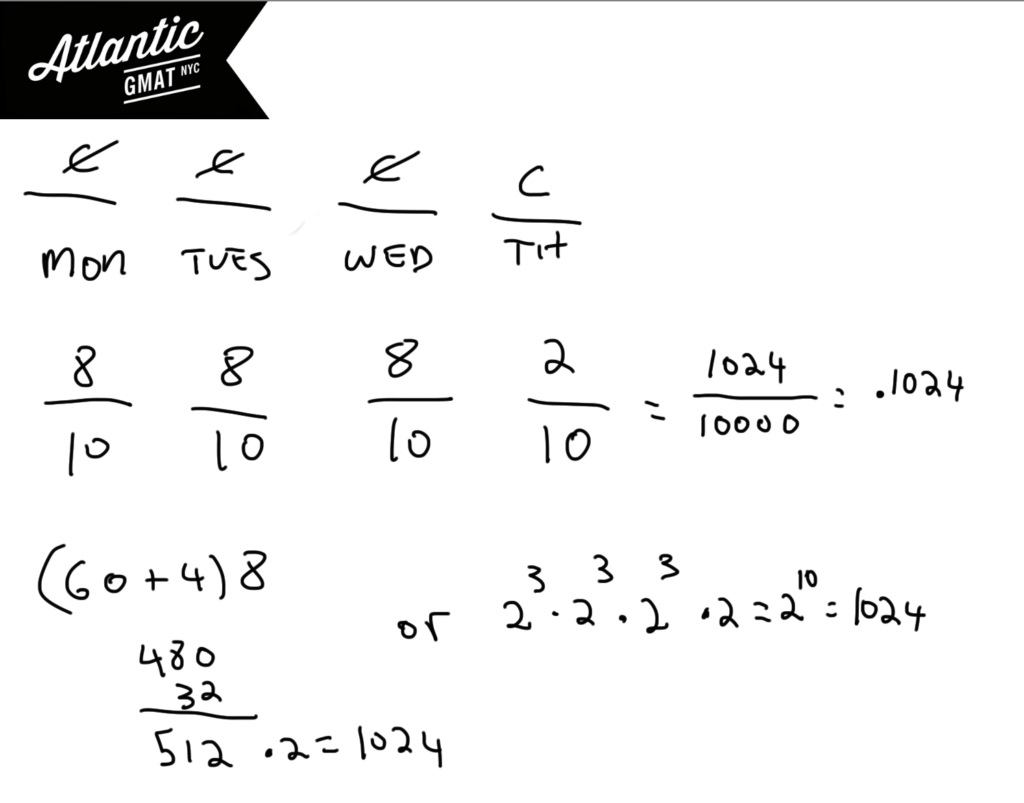# GMAT Question of the Day - Problem Solving - Probability

Katie has a bag of marbles which contains 3 blue marbles, 3 red marbles, and 2 yellow marbles. If she arranges her marbles in a line what is the probability that the marbles will be grouped by color?

A. 3/280

B. 3/560

C. 5/632

D. 7/632

E. 7/635

## GMAT Question of the Day Solution

GMAT probability can feel very easy or near impossible depending on your mastery of a few simple rules. Probability breaks down to:

Specific Scenario (in this case the marbles arranged by color)/Total Scenarios (in this case the total ways the marbles can arranged). It's important to realize that when calculating the total ways that the marbles can be arranged that switching two red marbles does not make a new arrangement (or switching two greens or switching two yellows). We need to use the non-unique ordering formula. The factorial of the total items (in this case 8 marbles so 8!) divided by the factorial of each individual item (3!, 3!, 2!). Any time that you are arranging/ordering non-unique items you need to use this formula.

Also realize that with the specific scenario the real question is: how many ways can you arrange three different colored marbles? Why? Well, you have to group each color so really each group just represents a color. Arranging three unique things is just 3!

Quick note on arithmetic. Notice in the solution that I didn't multiply 8, 7, 5, and 2 but waited until the very end so that I could simplify more. On the GMAT it's helpful to wait on multiplication until you are sure that you won't be able to simplify further. The GMAT usually gives you a way to keep your arithmetic manageable. Get in the habit of picking up on these cues.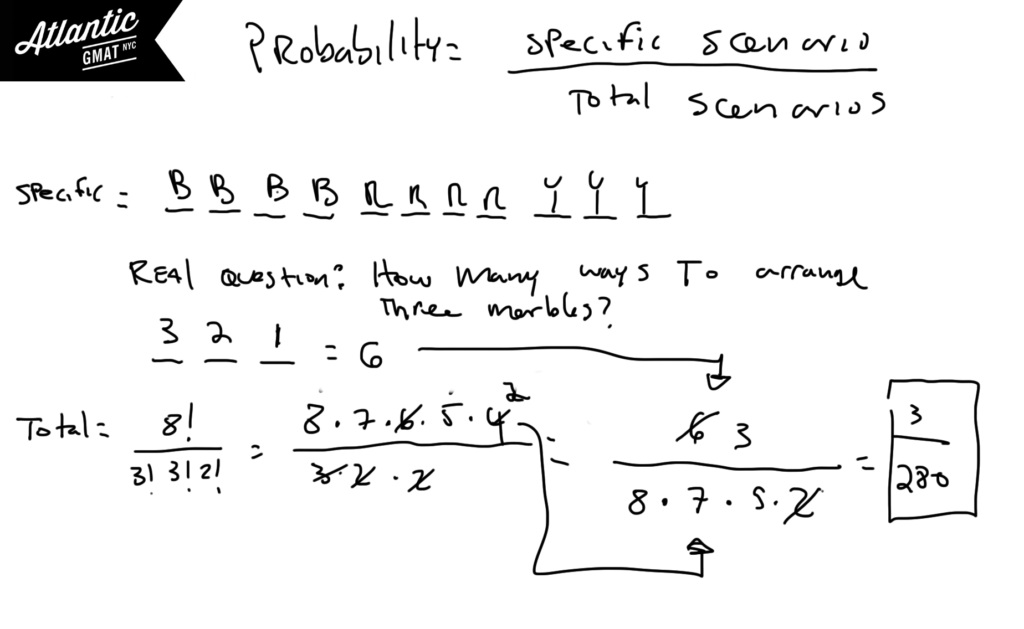# CONTACT

Atlantic GMAT

405 East 51st St.

NY, NY 10022

(347) 669-3545

info@AtlanticGMAT.com